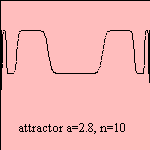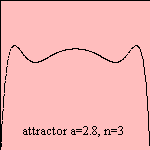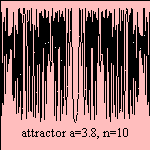# recurrent polynomial

## polynomial

last updated: 2003-08-13

It's also possible to define a polynomial with a recurrent method, given a starting function. There are an infinite number of polynomials y=f(x), where the nth is to derived from former ones:

## Fibonacci polynomialwith y0(x)=0, y1(x)=1
Named to Fibonacci, famous of his Fibonacci series.

## attractorwith ya,0(x) = x

Above formula states the iteration of a parabolic function, giving a polynomial of degree 2n. For 0 < a < 4 convergence to one or more values may happen. Such points of convergence 1) are called attractors, later on this term was also given to the iterated curve.The character of these patterns depends strongly on the value of the parameter a. For small values for parameter a, one sees just one point of convergence. Enlarging parameter a, at a certain moment a doubling of attractors is to be seen, the point of convergence seems to split, and later on again. Above some critical value 2) no separate attractors are to be distinguished, a chaotic region is entered.
In fact we're looking at polynomials of a rather high degree 3), on a small part of the domain. It appears that sometimes the generated polynomials have regions, where the function values are rather constant. Sometimes the extremities are so close to each other, that it resembles to chaos. This pattern changes with the parameter a.

The relationship can be used in population dynamics as a model (the Verhulst model) to describe a retained growth, for insects or other creatures. The parameter a is a measure of the fertility, for small values the species dies out. But there is also a maximum population size, caused by the term 1 - f.The physicist Mitchell Feigenbaum discovered that the quality of the maximum determines the behavior on the long term. It appeared that parabolic behavior is not necessary parabolic, a quadratic maximum satisfies. Efforts are undertaken to bridge the behavior of these curves to the chaos of the physical turbulence (until now, without real success) 4).
These phenomena were quite popular in the beginning of the 20th century. From the 70s on there is a revival, what produced a large stream of publications, especially in relation to chaotic behavior. This renewed attention is partly understood by the easier access to computing facilities, with whom the iterations can be easily calculated.

From these set of curves the fractal parabola bifurcation can be derived.

notes

1) The attractor point will always lay on the line y=x.